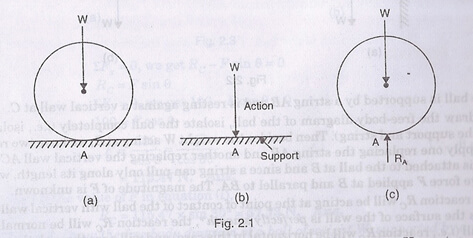Equilibrium of any stationary body occurs when external forces, either concurrent or parallel forces are to act upon a rigid body but no movement or rotation is resulted as its reaction. In that position the body is said to be in equilibrium. In this chapter you are going to find:

• Conditions of equilibrium for the external forces both concurrent and parallel separately.
• Moment of force on a point and in an axis.
• Varignon’s theorem.
• Free body diagram.
• Vectorial representation of moments and couples.
• Many types of support reactions.
• And what is the determination of reaction will be explained.

In the next portion, equations of equilibrium will be focused, such as:

• Equations of equilibrium: When studying equilibrium of rigid body, then there will be mentions on coplanar forces or as it is explained as concurrent and parallel forces. In the equilibrium state of the stationary body all mathematical outcome of external forces will become zero with the moments about the point and axis. The equation will be written as F = 0 and M = 0. The first equation is known as force law of equilibrium and the second one is known as the moment law of equilibrium. The forces are generally resolved into horizontal and vertical components. Hence equation (first) is written as Fx = 0 Fy= 0 whereFx= Algebraic sum of all horizontal components andFy= Algebraic sum of all vertical components.
• Equation for non-concurrent forces: This equilibrium occurs when all other forces and moments reach a mathematical result of zero. The equation is Fx = 0, Fy = 0 and M = 0.
• Equation for Concurrent forces: In this equilibrium all lines of actions are found in one point and so ultimately making moments of those forces also zero. Here M = 0 becomes unnecessary and the important equations remain as in Fx= 0 and Fy= 0.
• Action and reaction: In the third law of Newton, all actions have an equal amount of opposite reaction. This is an equal power of opposite features. In the figure below there are two balls placed horizontally. They can move freely horizontally but not vertically down. The ball will place an action which wants to move downward. The support will place an equal amount of opposite force to stop that ball to go downward than is calld reaction.Links of Previous Main Topic:-

Link of Next Mechanical Engineering Topics:-

Free body diagram in equilibrium of rigid bodies

### Submit Your Assignment### Customer Reviews

My Homework Help
Rated 5.0 out of 5 based on 510 customer reviews at